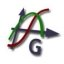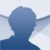# Graph

4.4.2.543

## Graph is a free application that allows you to draw maths graphs on a coordinate system. Download Graph and you will easily be able to draw functions

8/10 (24 Votes)

Any student or mathematician that needs to draw a function, coordinates or any kind of mathematical representation will surely use Graph. Once the program has been launched, all we will have to do is add the functions and calculations that we want to see represented.

### Represent math equations graphically

To show all the representations the software divides its interface in two, an area where it will show all the functions and mathematical expressions, and another in which we will see its representation on a coordinates axis.

With Graph, it is possible to add all kinds of representations: trigonometry, logarithmic, hyperbolic, etc. Of course, it also includes the possibility to show common maths constants like pi.

To sum up, Graph is a relatively complete software application in which it won't be complicated to obtain the graph of a function to help us understand it, furthermore, since we will instantly be able to modify it, we will be able to understand how they work a lot easier, no matter how complicated they are.

Ivan Johansen
Over a year ago
04/19/2021
9.6 MB

#### We'd love to hear from you. Do you want to give us your opinion?Logged off